Appendix 6 The Concept of Experimental Error and its Statistical Basis

To understand what is meant by the term "error" in the context of experimental measurement, consider the first experiment you did in C10J practicals - the determination of the acetic acid content of a sample of vinegar. Let's say you obtained a value of 4.79%. It is quite reasonable to ask of you (and your instructor who designed the experiment) how sure you both are that this result is the true value. How likely is it that you would obtained this same exact value again, if you repeated the experiment on a similar sample of vinegar, using very similar volume measuring devices, and using the same technique?

The answer of course is that it is very likely that you would not obtain this exact same value, even if you repeated it several times. A sceptical fellow student (especially one who is doing some philosophy) might well ask what good is your measurement if it is not likely to give the same result consistently. The truth is that if you repeated the measurement several times, you are likely to obtain values like those listed below:

 4.89 4.95 4.76 4.69 4.85 4.63 4.78 4.75 4.74 4.86 4.79 4.8 4.66 4.8 4.75

(These values were actually obtained by members of a former C10J class). One can see that the numbers are not just totally random - they range in value from 4.63 to 4.95, and are really not so very different at all from the single value you obtained. In fact if we were to collect all 50 values obtained by your classmates any one day, and plot a frequency distribution of the values, in groups, we are likely to obtain a plot similar to that shown below.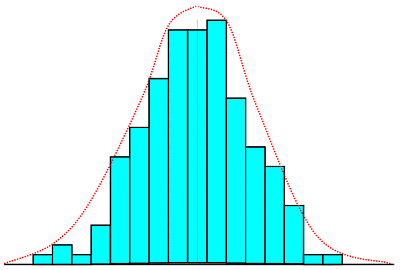The bar graph shows a typical distribution of say 50-odd data points, while the dashed line shows the distribution likely with large number of data points plotted in very narrow-width groups.

(A frequency distribution is the plot of the number of times a value occurs within a group, say 4.75 - 4.85, plotted against the group value). As you can see, this results in a fairly symmetrical pattern of distribution of values about a central value. The distribution is one which is fairly well known to statisticians. It is known as a Gaussian or normal distribution, but what is more important for us, is that it has properties which tell us quite a bit about the likelihood of obtaining certain values for our measurement, and that is exactly what we want to know.

When the results of repeated similar experiments follow a normal distribution we know that the most probable value of the quantity we are measuring is the mean (or average) value. We find also that 95% of the values obtained by repeating the experiment will fall between (the mean - 2σ) and (the mean + 2σ) where s is a measure of the broadness of the distribution. Also, 68% of the values can be expected to fall between (the mean - σ) and (the mean + σ).

The quantity σ is a measure of dispersion used by statisticians, and it is knows officially as the standard deviation or the standard error of the set of data. The formula for calculating σ is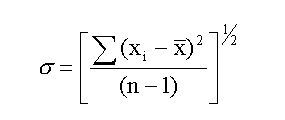The denominator, (n-1) is used instead of n when the sample is small (< 30). xbar is the mean value and (xi - xbar) is the deviation of each data point from the mean.

Applying this formula to the 15 data points listed earlier gives a standard deviation of 0.085, with the mean value being 4.78. The meaning of this result can be stated thus:
"95% of the values obtained by students in the lab group from which the sample is drawn, are likely to fall between 4.61 and 4.95, i.e. (4.78 ± 0.17) and 68% are likely to fall between 4.69 and 4.87, i.e. (4.78 ± 0.09)."

We can view this another way. For any single determination of x, there is a 95% probability that the mean will fall between (x - 2s) and (x + 2s) and 68% that it will fall between (x - s) and (x + s). When it is viewed this way , it is obvious that s is a measure of the error (or uncertainty) associated with the experimental determination of x. But what should we write as the error, ±s or ±2s? We can write either, as long as we make it clear which one is being written. The latter (±2s) is quite a conservative estimate and even the former (±s) represents better than 50% certainty that the mean falls within the range specified. (The value which represents 50% certainty is in fact known as the probable error and it turns out to be ±0.67s). For reasons which we will see later, it is it is the most probable error that is usually written as the error in measurements. However it does not make a great deal of difference if we regard the error as ±s.

So, if we had known the value of σ, we could have written the result of our single measurement as:
acid content = (4.79 ±0.09)%

The result could then have been interpreted (to your philosophical fellow student, for example), to mean that if you had repeated the experiment several times using the same equipment and technique, the average of these repeated determinations is very likely to fall within the range 4.70 to 4.88.

One begins to really appreciate the importance of this statement of error, when one is asked to make a judgement on the value obtained for the acid content of vinegar. Suppose, for example, that the label on the product, as it is sold on the market, claims that the acid content is 6.5%, could we use your single result as the basis for saying that the producer has labelled the this product wrongly? The answer would of course be yes. For if we know the value of s to be 0.085, we are quite confident (95%) that the acid content is somewhere within the range 4.61 to 4.95.

We might wish in fact to improve our confidence in the value, by doing the determination once or twice again, using the mean of these determinations instead of the single value, as our experimental result. We might also wish to check that our reagents were really as pure as we assumed, and that our standard NaOH was indeed the molarity stated, before we made any public accusations. It should be quite clear however that we can make judgements based on our results, provided we know what uncertainty or error we can reasonably associate with these results. Conversely it would be fair to say that your result has no real use or meaning if you have no idea of the uncertainty associated with that result.

Types of Error, Accuracy and Precision
It should be evident from our discussion so far that experimental errors exist and are present (to some extent) in all experimental measurement. Our task as experimental scientists must therefore be to design experiments to produce the best results we can, in spite of such errors, and to assess the uncertainty which these unavoidable errors cause in our measurement.

It is useful to distinguish between two types of experimental error - systematic (or bias) error, and random error, though the distinction can appear to be somewhat artificial in some cases.
Systematic error is experimental error whose numerical value tends to remain constant, or follow a consistent pattern over a number of experimental runs. In the determination of the acid content of vinegar, systematic error would have been introduced if the standard sodium hydroxide was of a lower molarity than it is said to be. Suppose its molarity was determined from the mass of NaOH pellets made up to say 500 cm3. This, apart from being bad practice, would almost certainly introduce systematic error into the determination because NaOH pellets absorb water from the atmosphere very rapidly, when they are being weighed.

The mass of water absorbed would have been interpreted as mass of NaOH and so the true molarity would have been less than that calculated. The molarity of acid determined by titration against this base would turn out to be systematically greater than the true value.

We can generalize a bit and say that systematic error arises from incorrect procedure, incorrect use of instruments, or failure of some value to be what it is assumed to be.

Random error, on the other hand, is experimental error whose numerical value and sign are likely to change from one measurement to the next. The average value for random error is zero, and it is the random error in measurements which cause the frequency distribution of repeated experimental results to follow the normal or Gaussian distribution mentioned earlier.

The error which arises because one does not have ones eyes exactly level with the bottom of the meniscus, when reading a burette, is usually random error. Sometimes the eyes will be above the level and the reading will be greater than the true value, while some times the eyes will be below the level and the reading will be less than the true value (usually by small amounts). Many of you already have a "rule of thumb" for estimating random error in readings such as that of a burette - the error being approximately ± a half of the smallest division. This is a good estimate in some cases, but not so good in others, as is illustrated below: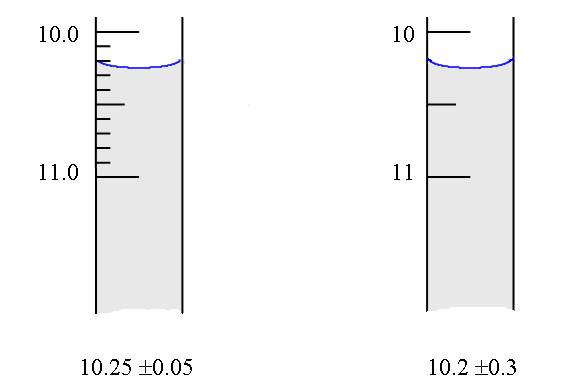These two types of errors usually have very different effects on the results of an experiment. To illustrate this, we took 30 results of students doing the determination of the acid content of vinegar on a different lab day from those already given. These results had a mean of 4.57 and a standard deviation of 0.068, (compared to 4.78 and 0.066). The distribution from which these samples are drawn can be represented by the two curves shown below.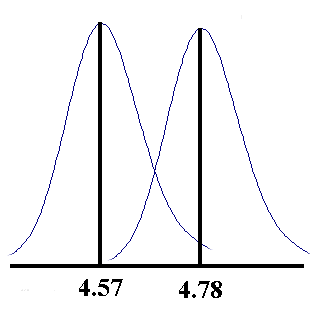Systematic errors account for the shift in the mean from one day to the next, while random errors account for the spread of the values about the mean each day. The random error can be seen to be associated more with the technique and instrumentation used, from the fact that it is about the same each day the experiment is done.

What do you think is the cause of the systematic difference between the results? We say that systematic error affects the accuracy of the result - i.e. its closeness to the 'true value, while ramdom error affects the precision or repeatability of the results. The 'true' value is simply the best available experimental value, in our case. For universal constants such as R, the gas constant, and h, Plank's constant, the true or accepted value is the mean of the values obtained by the best experiments done by different workers.

Coping with Errors in Measurement
Systematic errors can be identified and their effect reduced, by putting some thought into the design of the experiment, to ensure in particular that experimental conditions are controlled, the equipment is appropriately calibrated and that any assumptions which are inherent in the technique, are adequately met in the conditions selected.

In the experiment to determine the acid content of vinegar, good practice would require that each batch of "standard" NaOH is standardized against a good reproducible standard such as pure potassium hydrogen phthalate, and that an indicator which changes near the equivalence point (pH » 8.0) is used. This ensures that the assumption that the acid and base are present in equivalent number of moles, is valid in our experiment. These and other features of the design which are aimed specifically at reducing systematic error are usually not recognized by those who follow the instructions in recipe-like fashion. But how does one decide which of the two lab days had the more accurate result?

If all else fails, a good check on the accuracy of the result would be to have the determination done independently, elsewhere - perhaps by a completely different method in a non-University lab. Agreement between two such independent determinations is very strong support for any claim of accuracy. When it is not possible to have such independent checks done, "internal" monitoring of accuracy can be achieved by determining an accurately known standard of acetic acid with each new batch of reagents used, and by determining this standard as part of the routine on each lab day.

The strategy for dealing with random error is some what different, for it relies mainly on repeating the same experiment a number of times. The rationale for this approach is simply the fact that the average of a number of observations is more precise than a single observation. In the acetic acid determination the mean of 33 values, 4.78, is more precise than the single value obtained by any individual. The standard error in the mean is given by the statistical considerations as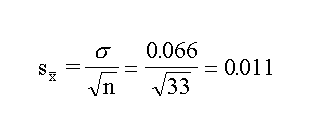So whereas the single value has a standard error of 0.066, the mean has a standard error of 0.011.

Since the value of 1/√n decreases very rapidly for increasing n, when n is small, it is good practice to repeat the determination a couple of times, if you want to be a bit more confident about your results. That is why it was recommended earlier that you repeat the determination of acetic acid in vinegar at least once or twice before making accusations against the manufacturer.

Medical laboratories usually do their determinations in duplicate or triplicate for this reason, and many of the run "internal" standards with each batch of samples, as a means of checking on changes in the accuracy of the procedure from week to week.

Of course it follows that more precisely calibrated instruments will help to improve precision. A thermometer which has its smallest division as 1°C is likely to have reading errors of about ±0.5°C, (i.e. half of the smallest division), while one with ten divisions per 1°C can be read to about ±0.05°C.

Lastly it should be pointed out that you do not need to have the most accurate and most precise instruments available to do every measurement. For example it is usually sufficient to determine the melting point of a substance to ± a tenth of a degree Celcius to ascertain its purity for most practical purposes in Organic labs. But a thermometer is required which is fairly accurate, because it is the absolute value of the temperature on which the assessment of purity is based.

On the other hand the measurement of the temperature change which accompanies the dissolution of 1 g of potassium nitrate in 100 cm3 of water, (about 0.50°C), requires a more precise measurement, perhaps to ± a hundredth of a degree Celcius. The absolute accuracy of the thermometer is not all that critical because the assessment here is based on a temperature difference.

When you put 2 kg of water into the jacket of a bomb calorimeter, you need to know this with a precision of only ± 1 g to have a 0.05% precision, but when you weigh out 2 g of potassium phthalate to make up standard solutions you need to know the mass to ± 1 mg in order to have the same precision.

Estimating Error in a Single Determination

The random error which one should ascribe to an experimental result has been shown to be ± s. So far we have seen this standard error determined only from statistical analysis of a number of repeated determinations of the experimental result. The question of what to do when you have made only one or two determinations of the experimental quantity, is still unanswered. It is an important question because in most of our experiments we have time to determine the final value only once, even though we may do duplicate determinations of some primary quantities in the procedure.

We do already have some idea of the error associated with the measurement of individual primary quantities like volume, time and mass, (± ½ the smallest division), and it seems reasonable to assume that if we combine these errors, using the methods of statistics, we should end up with a good estimate of the error in the final result. In fact, most of you already make some estimate of error in your final result by taking the sum of the percentage errors in the various primary measurements. This is not quite correct from a statistical point of view. It is in fact the square root of the sum of the squares of the errors, or percentage (relative) errors that really represents the combine error.

The following rules can be used to give a reasonable estimate of the combined error in your final result.

(a) For sums and differences the actual error in the result is the square root of the sum of the squares of the actual errors in the contributing terms:

If,
A(±a) . B(±b) = C(±c)

where a is the actual error in A, etc. then
c = (a2 + b2)½

EXAMPLE        A titre volume which is usually obtained by taking the difference in burette readings before and after titration, will have a combined error of each reading (± 0.05 cm3) given by:

Final reading 18.30 ± 0.05 cm3
Initial reading 5.05 ± 0.05 cm3

Titre volume 13.25 ± ?

c = [(0.05)2 + (0.05)2]½ = 0.07 cm3

Titre volume = 13.25 ± 0.07 cm3

(b) For products and quotients the relative (or percentage) error in the results is the square root of the sum of the squares of the relative (or percentage) errors in the contributing terms.

If,
A(±a) x B(±b) = D(±d)

then,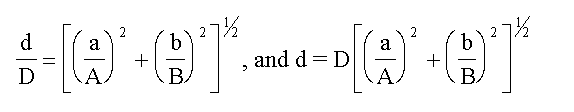EXAMPLE        Consider the case of the molarity of acetic acid determined from titration against standard NaOH.

Macid = (MNaOH. VNaOH) / Vacid

If we represent the error in each quantity as D(quantity), we haveAssuming that the error in the 10 cm3 pipette, used to take the sample of acid for titration, is about ± 0.05 cm3; and assuming negligible error in the molarity of NaOH, we have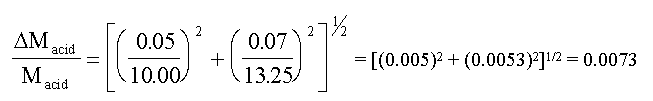So the relative error in the acid molarity is 0.0073 or 0.7% (using some actual data taken from a C10 student).
(c) To calculate the error in the final result, arrange the equation (used to get the result) in its simplest form, and combine errors using the rules given above. You may neglect any error (or a relative error) which is less than a tenth of the largest error (or relative error).

EXAMPLE
In the case of the acetic acid determination, we already have established in the above example that ~ 0.7% error exists in the molarity of the diluted acid due to uncertainty of the volumes used in titration. But there is also an uncertainty due to the fact that you took an aliquot of 10.00 ± 0.05 cm3 of the acid and diluted that up to 100.0 ± 0.2 cm3. Both uncertainties contribute to the error in the dilution factor resulting in an uncertainty of about 0.053 in 10.00, the error in the volume of the diluted acid being almost negligible.

The final result which is the mass of acetic acid in 100 g of vinegar is given by mA, where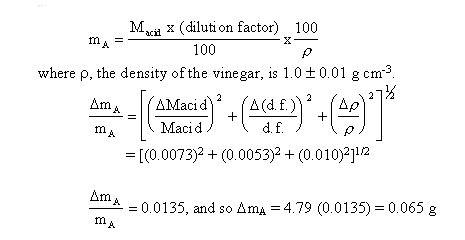We have estimated the error in our single value, 4.79, and found it to be approximately ± 0.065. This is really quite a good estimate considering that the value determined from the statistical spread of 33 repeated values was ± 0.066. This agreement between the two is better than what one would normally expect, but it does make the point that good estimates of error can be made for an experimental result by considering the error involved in the individual primary measurement.

It is this "art" which we hope you will develop. Accordingly we expect you to do an error analysis in every experiment you do in the Physical Chemistry Lab, and will treat it as an integral part of your report on lab-work for purposes of assessment.

Significant Figures

Every measurement should be recorded to as many figures as are significant, and no more. Wherever possible the uncertainty of the measurement should be recorded, but if it is not known, it is assumed to be uncertain by ± 1 in the last digit.

Those zeros in a number which locate the decimal point are not significant; 0.00314 and 80200 each have three significant figures. The exponential (scientific) notation, 8.0800 x 104 should be used.

The logarithm of a number should have as many significant figures in the mantissa as there are significant figures in the number.

Example        antilog 0.032 = 1.08. The result shows three significant figures in the mantissa.

Note that in the case of logarithms, the zero of the mantissa is counted.

The number of significant figures of a computed result is limited by the term with the largest uncertainty. In addition and subtraction, the number of significant figures in the result depends on the term with the largest absolute uncertainty.

Example:        1.9546 g + 2.03 g = 3.98 g. The result is expressed only to the nearest 0.01 g because 2.03 is only expressed to the nearest 0.01 g.

Example:        68.7 cm - 68.42 cm = 0.3 cm. This result is expressed only to the nearest 0.1 cm because 68.7 cm is only expressed to the nearest 0.1 cm.

In multiplication and division, the number of significant figures in the result depends on the term with the largest relative uncertainty. The relative uncertainty of a quantity is the absolute uncertainty divided by the value of the quantity.

Example:        0.26 cm x 21.902 cm2 = 5.7 cm3. The result is expressed to two significant figures because the relative uncertainty in 5.7 cm, 0.1/5.7 or 1.8%, is about the same as that in 0.26 cm, 0.01/0.26 or 3.8%. In multiplication and division the number of significant figures in the result is usually equal to that of the term with the least number of significant figures. In some cases, however, the result may have a greater number of significant figures than that of the term containing the least number of significant figures.

Example:        1049 g/94 g mol-1 = 11.2 mol and not 11 mol. The result is expressed to three significant figures rather than to two because the relative uncertainty of 11.2 mol, 0.1/11.2 or 0.9%, is about the same as that for 94 g mol-1, 1/94 or 1.1%, while that of 11 mol, 1/11 or 9.1%, is much larger.

In performing extended calculations, one should carry along one more than the allowed number of significant figures. When the answer is obtained, it can be rounded off to the correct number of significant figures.

In rounding off a number the following rules should be observed:

When a number smaller than 5 is dropped, the last remaining digit is left unchanged: when a number larger than 5 is dropped, the last remaining digit is increased by one digit: When the number to be dropped is a 5 followed by a 0, the last remaining digit is rounded off to the nearest even digit.

Example:        The following numbers are rounded off to 3 figures: 1.7348 becomes 1.73; 1.735 becomes 1.74.Return to Chemistry, UWI-Mona, Home Page

Copyright © 1995-2009 by The Department of Chemistry UWI, Jamaica, all rights reserved.

Created and maintained by Prof. Robert J. Lancashire,
The Department of Chemistry, University of the West Indies,
Mona Campus, Kingston 7, Jamaica.
Created Mar 1995. Links checked and/or last modified 19th October 2009.
URL http://wwwchem.uwimona.edu.jm/lab_manuals/c10appendix6.html